# B. D. S., M. Sc. O. and Jassim Ali Jassim Al-E'nizy

 Page 3/4 Date conversion 16.05.2018 Size 0.93 Mb.
1   2   3   4

## Point Pog (Pogonion): The most anterior point of the bony chin in the median plane.

• Point Pt (Pterigoid): The anatomical point representing the foramen rotundum located at the junction of foramen rotendum with the upper region of the pterygomaxillary fissure.

• Point Dc (Condyle): The point in the center of the condylar neck where the Basion-Nasion plane croses it.

• Point Pm (Protuberance Menti): The point at the anterior border of the symphesis between point B and Pogonion where the curvature changes from concave to convex.

• Point Me (Menton): The lowest point in the symphyseal shadow of the mandible.

• Point Go (Gonion): the most posterior and inferior point at the angle of the mandible, where the bisector of the angle between tangents to the posterior and inferior borders of the mandible meets the mandibular outline.

• Point Gn (Gnathion): The most anterior and inferior point of the bony chin.

• Point Xi (at the Center of the Ramus): the location of this point is keyed geometrically to the Frankfort horizontal and pterygoid vertical planes.

Point Xi: This point located at the geographic center of the ramus and it could be determined by defining four major bony landmarks on the external border of the mandible and four lines figure(5) ,those are

1. R1-Mandible: the deepest point on the curve of the anterior border of the ramus, one-half the distance between the inferior and superior curves.

2. R2-Mandible: a point located on the posterior border of the ramus of the mandible opposing to R1.

3. R3-Mandible: a point located at the center and most inferior aspect of the sigmoid notch of the ramus of the mandible.

4. R4-Mandible: a point on the lower border of the mandible directly inferior to the center of the sigmoid notch of the ramus, opposite to R3.

5. A Line Tangent to the Anterior Border of the Ramus at R1 perpendicular to Frankfort plane and parallel to the pterygoid vertical plane.

6. A Line Tangent to the Posterior Border of the Ramus at R2 perpendicular to Frankfort plane and parallel to the pterygoid vertical plane.

7. A Line Tangent to the Superior Aspect of the Mandible at point R3 parallel to Frankfort plane and perpendicular to the pterygoid vertical plane.

8. A Line Was Done at the Lower Aspect of the Mandible parallel to the Frankfort plane and perpendicular to the pterygoid vertical plane at point R4. The intersections of these lines would produce a rectangle representing the entire ramus, from each two contralateral angles a plane can be drawn, the intersection between these two planes can represent the Xi point (RMO, 2000;Al-Tamimy, 2006)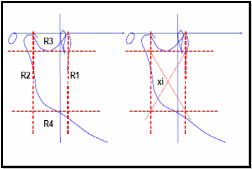Figure (5) Diagrammatic Detection of Xi Point in the Mandible (RMO, 2000)

Frontal Cephalometric Landmarks:

The following landmarks were used in this study

1. Point Zy (Zygomatic Arch): At the most lateral border of the center of the root of the zygomatic arch (right and left).

2. Point Nasion: The most anterior point of nasofrontal suture in the midsagittal plane.

3. Point Menton: The most caudal point in the outline of the symphesis, it is regarded as the lowest point of the mandible.

Angular Measurements:

For describing the face Ricketts used five angles. These five angles are:

1. The facial axis angle (Ba-N-Pt-Gn lines) which is formed between the facial axis or the central axis of the face and the Basion-Nasion plane .This angle gives information about the chin.

2. The facial angle (FH-N-Pog) which is formed between facial plane (N-Pog) and (FH) plane. This provides some indication of mandibular prognathism.

3. The lower facial height angle (ANS-Xi-Xi-pm) which is formed by intersection of a line from anterior nasal spine to the center of the ramus (the Xi point) and the corpus axis (from the center of the ramus to the supra pogonion(pm)). It gives an indication of skeletal open bite.

4. The mandibular plane angle (FH-MP) which is formed between frankfort horizontal and mandibular planes. It was used as an indicator of skeletal morphology of the mandible.

5. The mandibular bend angle (DcXi-XiPm): measures the angulation of the condylar process to the body of the mandible.

Frontal Linear Measurements:

Linear measurements (2 Skeletal) were recorded:

1. Zy-Zy: This represents the facial width or interzygomatic distance, measured from left Zy to right Zy.

Total Anterior Facial Hight (Nasion –Menton); this represents the total anterior facial height. It was measured as the direct distance from nasion to menton.

Dental Arch Forms:

For determination of the dental arch form, the method used by standardization, six dental cast’s measurements were divided into three sagittal measurements, and three transverse measurements were utilized to calculate three independent ratios, which are:

• Anterior arch length (canine vertical distance) / inter-canine distance.

• Molar vertical distance / inter-first molar distance.

• Total arch length / inter-second molar distance.

For each ratio, the average was calculated for the maxillary dental arches for each sex, then standardization was done for each of three ratios for each subject by the excel program. Then the mean of these standardized numbers was calculated for each subject which gave the base for classification as fellows:

Form 1, (Narrow) the three sagittal /transverse ratios are positive (greater than the mean).the mean of standardized number >+1.

Form 2, (Mid) none of the ratios significantly deviated from the average. the mean of standardized number between (+1 and -1).

Form 3, (Wide) the three sagittal /transverse ratios are negative (lesser than the mean). The mean of standardized number <-1.
Lateral Facial Type

In this study the mean of standardization of each five values of the person was calculated for each gender (by standardization compare the values with the mean according to the standard deviation) The standardized numbers of the facial axis angle, the facial angle, the mandibular bend angle multiplied by (-1).So that the dolichfacial pattern would have a mean of standardized number more than (+1), the brachyfacial had a mean of standardized number less than (-1) and the mesofacial had a mean of standardized number between (-1 and +1).

Frontal Facial Type: Determined facial types by calculating the ratio between interzygomatic distance and total anterior facial height then the face type for each subject is classified as follows;

• Euryprosopic

Facial Index=N-Me (height)/ IZD (Bizygomatic breadth)

• Mesoprosopic

Facial Index= N-Me (height)/ IZD (Bizygomatic breadth) with in average

• Leptoprosopic

Facial Index= N-Me (height)/ IZD (Bizygomatic breadth) > average.

By standardization the facial index the person that has standardized number more than (+1) would be Leptoprosopic facial type, the person that has standardized number less than (-1)would be Euryprosopic facial type and the person that has standardized number between (+1 and -1) would be Mesoprosopic facial type (Cakirer et al, 2001).

Statistical Analysis:

The data recorded in this research were subjected to computerized statistical analysis using both Excel-2007, and SPSS version 15 programs

The statistical analysis included

1.Descriptive Statistics (Mean, standard deviation, minimum, maximum) for all facial measurements angles, liner and ratios taken from the (lateral and frontal)digital cephalogram, and also for the maxillary dental arch widths, lengths and ratios for the sample.

t-test” was applied to test the significant differences between the mean of all dimensions of upper dental arches &facial measurements in Male and Female.
2- Standardized number of the five angles (from lateral cephalometric)and ratio of the total anterior facial height/interzygomatic distance (from frontal cephalometric).

3- Standardized number of three ratios(from study models).

• Anterior arch length/inter-canine distance.

• Molar-vertical distance/inter-first molar distance.

• Total arch length/inter-second molar distance.

RESULTS

Dental Arches:

Description and Comparison between Males and Females:

All the variables of the maxillary dental arch widths and, T-test was applied to see if there were any differences between males and females. It revealed that the males had larger arch width with a highly significant difference at P < 0.01 in all width dimensions in maxillary arches as illustrated in Table (1) and Figure (6).

for maxillary dental arch lengths. The statistical analysis showed that there were high significant differences between males and females in maxillary vertical distance, all of the vertical measurements showed that males had larger arch length than females with a high significant difference at P < 0.01 Table (2) and Figure (7).

Table (1) Descriptive Statistics for Maxillary Arch Width with Comparisons between the Genders.
 Variable sex Mean ± SD Min Max t-test Inter Canine M 36.19 1.91 32.58 41.18 5.450** F 34.11 1.73 30.9 38.52 T 34.92 2.06 30.90 41.18 Inter 1st Molar M 55.96 2.83 49.56 61.7 5.658** F 52.93 2.31 47.62 59.44 T 54.11 2.92 47.62 61.7 Inter 2nd Molar D M 60.07 2.74 53.17 64.78 6.293** F 56.58 2.54 52.34 63.29 T 57.94 3.12 52.34 64.78

NS. : Non Significant at P > 0.05. ** : Significant at P < 0.01. All dimensions in mm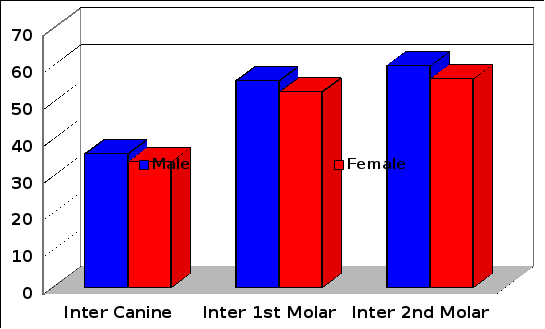Maxillary dental arch width (mm)

Figure (6) Mean of Maxillary Dental Arch Width for both Genders.

Table (2) Descriptive Statistics for Maxillary Arch Length with Comparison between the Genders.
 Variable Sex Mean ± S.D Min. Max. t-value Anterior Arch Length M F T 8.81 8.31 8.50 0.90 0.83 0.89 6.67 6.56 6.56 10.68 10.35 10.68 2.790** Molar Vertical D M F T 30.09 28.51 29.13 1.56 1.70 1.81 26.57 24.34 24.34 33.85 33.61 33.85 4.560** Total Arch Length M F T 44.86 42.37 43.34 1.98 1.83 2.24 40.71 37.53 37.53 48.75 47.31 48.75 6.267**

NS : Non Significant at P > 0.05. ** : Significant at P < 0.01. All dimensions in mm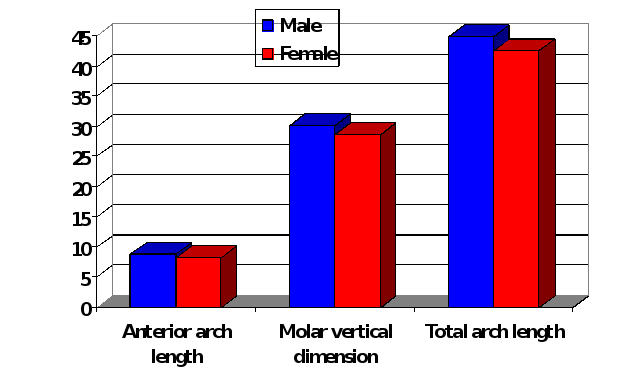Figure (7) Mean of Maxillary Dental Arch Length for both Genders.

Arch Form:

Table (3) and Figure (8) showed the average of the three ratios used in the determination of the dental arch forms (Anterior arch length/inter-canine distance; molar-vertical distance/inter-first molar distance, total arch length/inter-second molar distance) for the maxillary dental arches. there was no significant difference between males and females in the three ratios.

Table (4) and Figure (9) showed the distribution of the three forms of maxillary dental arch, It could be noticed that the most prevalent arch form among the sample was the Mid form (54,05%, 55,17% and 54.73%) followed by the Narrow form (24,32%, 24,13% and 24.21%), while Wide forms being (21,62%, 20,68% and 21.05%) for males, females and the total sample respectively .
Table (3) Description for the Three Ratios for (Males and Females) with Comparisons between the Genders.

 Sex Ratio Sex Mean Sd. Min Max T-Value Canine Vertical / Inter Canine M F 0.244 0.244 0.0269 0.0236 0.193 0.180 0.313 0.292 0.023NS Molar Vertical / Inter 1st Molar M F 0.5430 0.5340 0.0303 0.0266 0.491 0.485 0.622 0.624 0.342NS Total Arch Length / Inter 2nd Molar M F 0.747 0.749 0.0294 0.0278 0.669 0.629 0.845 0.827 0.162NS

NS: Non Significant at P > 0.05.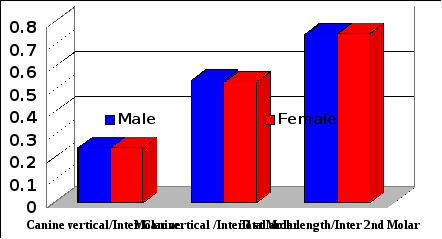Figure (8) The Three Ratios for (Males and Females) with Comparisons between the Genders.

Table (4) Distribution of the Three Maxillary Arch Forms for (males,females and the Total) (Frequencies and Percentages).
 Males Females Total Arch Type No. % No. % No. % Narrow 9 24.32 14 24.13 23 24.21 Mid 20 54.05 32 55.17 52 54.73 Wide 8 21.62 12 20.68 20 21.05 Total 37 100 58 100 95 100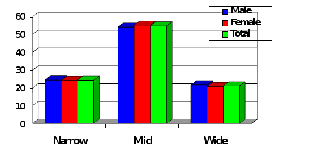Figure (9) Percentages of Three Maxillary Arch Forms for(Males, Females and the Total).
• 1   2   3   4

The database is protected by copyright ©dentisty.org 2016
send message

Main page